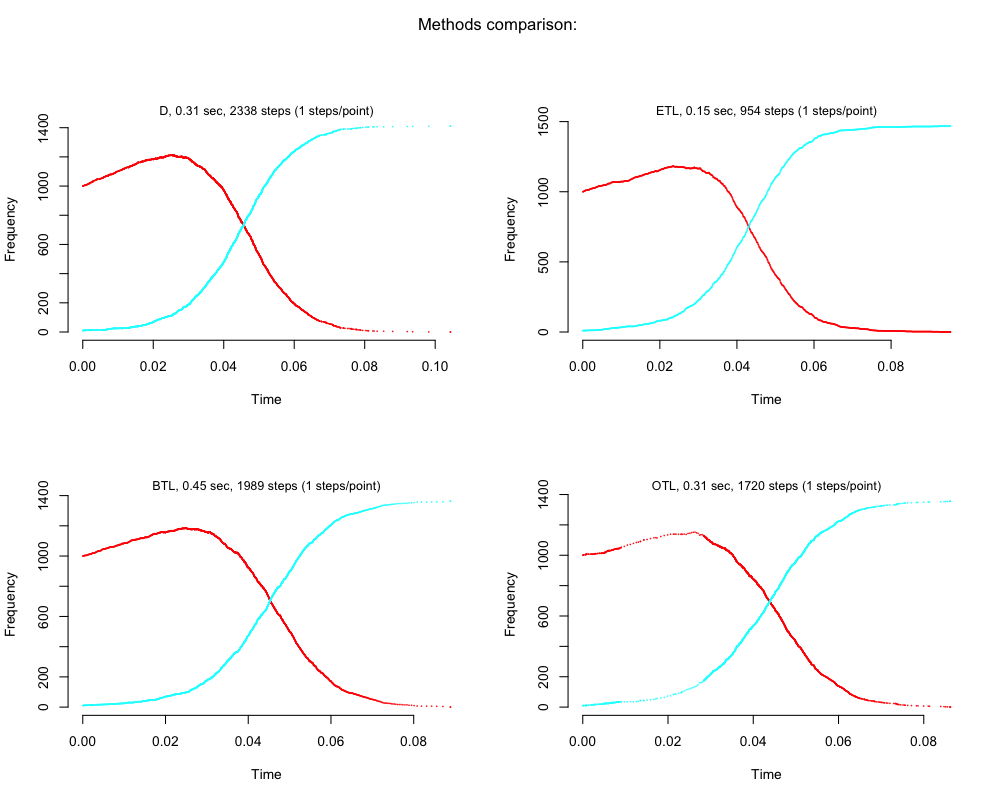# Zombie predator-prey model

### Because zombies are trendy in 2013Implementation with GillespieSSA: Gillespie's Stochastic Simulation Algorithm (SSA)

It is a prey-predator model zombified: prey became a predator when die, no reproduciton for zombies

## The Model (Zombie Lotka-Volterra)

1. Human reproduce: $$h+h \xrightarrow{c_1} h+h+h$$
2. Humans can die of natural death: $$h \xrightarrow{c_2} 0$$
3. Humans can die for a zombie attack: $$z+h \xrightarrow{c_3} z+z$$
4. Zombies can be killed by heroic humans: $$h+z \xrightarrow{c_4} h$$

Differential equations:

Humans: $$\frac{\delta h}{\delta t} = c_1 * h * h - (c_2 * h + c_3 * h * z)$$

Zombies: $$\frac{\delta z}{\delta t} = c_3 * h * z - c_4 * h * z$$

### Propensity vector

The propensity vector a defines the probabilities that a particular reaction will occur over the next infinitesimal time interval $$[t, t + dt]$$ . The propensity vector consists of character elements of each reaction's propensity function where each state variable requires the corresponding named element label in the initial state vector (x0).

a <- c("c1*h*h", "c2*h", "c3*h*z", "c4*h*z")


### Initial state vector

The initial state vector defines the population sizes in all the states at $$t=0$$.

x0 <- c(h = 1000, z = 10)


### State change matrix

The state-change matrix nu defines the change in the number of individuals in each state (rows) as caused by one reaction of a given type (columns).

nu <- matrix(c(+1, -1, -1, 0, 0, 0, 1, -1), nrow = 2, byrow = T)

c1 c2 c3 c4
h 1.00 -1.00 -1.00 0.00
z 0.00 0.00 1.00 -1.00

Where $$c_1$$, $$c_2$$ $$c_3$$ and $$c_4$$ are the per capita reaction probabilities and $$h$$ and $$z$$ are human and zombies.

### Running the simulation

Define parameters:

params <- c(c1 = 0.01, c2 = 0.1, c3 = 0.1, c4 = 0.01)


Set a seed, a final time and a name for the simulation:

set.seed(1)
tf <- 10000
simName <- "Zombies outbreak"


#### Direct method

out.d <- ssa(x0, a, nu, params, tf, method = "D", simName = simName, verbose = TRUE,
consoleInterval = 1)

## Running D method with console output every 1 time step
## Start wall time: 2013-09-05 16:05:51...
## t=0 : 1000,10
## t=0.1043 : 0,1411
## tf: 0.1043
## TerminationStatus: zeroProp
## Duration: 0.315 seconds
## Method: D
## Nr of steps: 2338
## Mean step size: 4.462e-05+/-0.0002026
## End wall time: 2013-09-05 16:05:52
## --------------------

ssa.plot(out.d)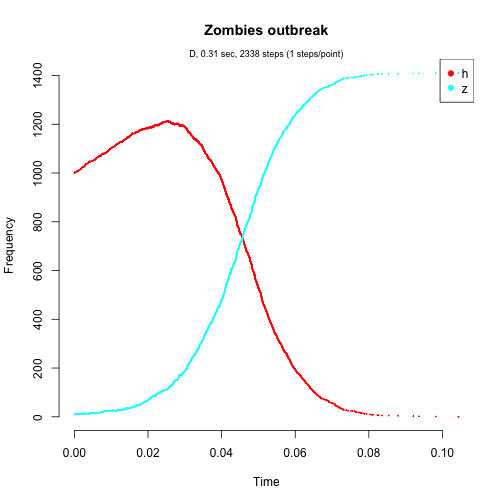#### Explict tau-leap method

out.e <- ssa(x0, a, nu, params, tf, method = "ETL", tau = 1e-04, simName = simName,
verbose = TRUE, consoleInterval = 1)

## Running ETL method with console output every 1 time step
## Start wall time: 2013-09-05 16:05:52...
## t=0 : 1000,10
## t=0.0954 : 0,1468
## tf: 0.0954
## TerminationStatus: zeroProp
## Duration: 0.146 seconds
## Method: ETL
## Nr of steps: 954
## Mean step size: 1e-04+/-0
## End wall time: 2013-09-05 16:05:52
## --------------------

ssa.plot(out.e)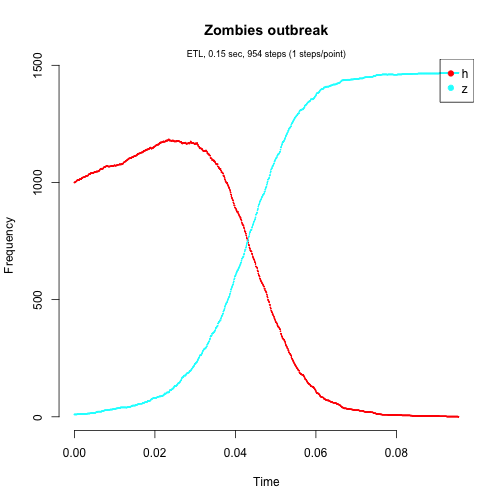#### Binomial tau-leap method

out.b <- ssa(x0, a, nu, params, tf, method = "BTL", f = 1.1, simName = simName,
verbose = TRUE, consoleInterval = 1)

## Running BTL method with console output every 1 time step
## Start wall time: 2013-09-05 16:05:52...
## t=0 : 1000,10
## t=0.08888 : 0,1363
## tf: 0.08888
## TerminationStatus: zeroProp
## Duration: 0.454 seconds
## Method: BTL
## Nr of steps: 1989
## Mean step size: 4.468e-05+/-8.654e-05
## End wall time: 2013-09-05 16:05:52
## --------------------

ssa.plot(out.b)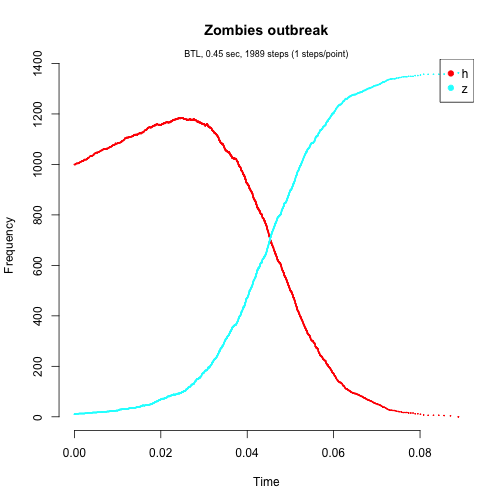#### Optimized tau-leap method

out.o <- ssa(x0, a, nu, params, tf, method = "OTL", epsilon = 0.01, nc = 100,
hor = NaN, dtf = 10, nd = 100, simName = simName, verbose = TRUE, consoleInterval = 1)

## Running OTL method with console output every 1 time step
## Start wall time: 2013-09-05 16:05:52...
## t=0 : 1000,10
## t=0.08626 : 0,1356
## tf: 0.08626
## TerminationStatus: zeroProp
## Duration: 0.315 seconds
## Method: OTL
## Nr of steps: 1720
## Mean step size: 5.015e-05+/-0.0001278
## Nr suspended tau leaps: 1600(100%)
## End wall time: 2013-09-05 16:05:53
## --------------------

ssa.plot(out.o)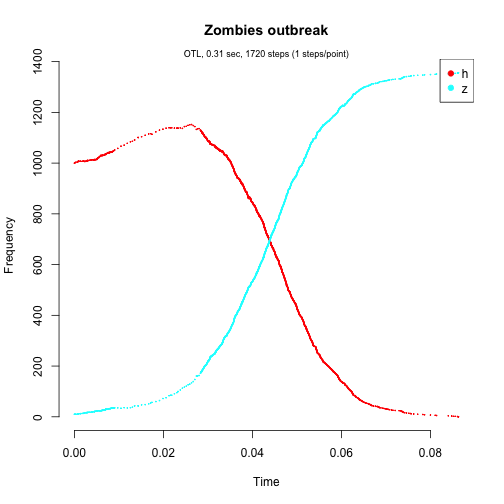#### Compare results (using a layout)

Here we use some figure knitr chunk options (check source code):

1. dots per inch: dpi=100
2. fig sizes (inches): fig.width=10, fig.height=8
3. fig output sizes: out.width=“800px”, out.height=“600px”